# Question 1 Question 2 Question 3 Describe the

• Slides: 30Question 1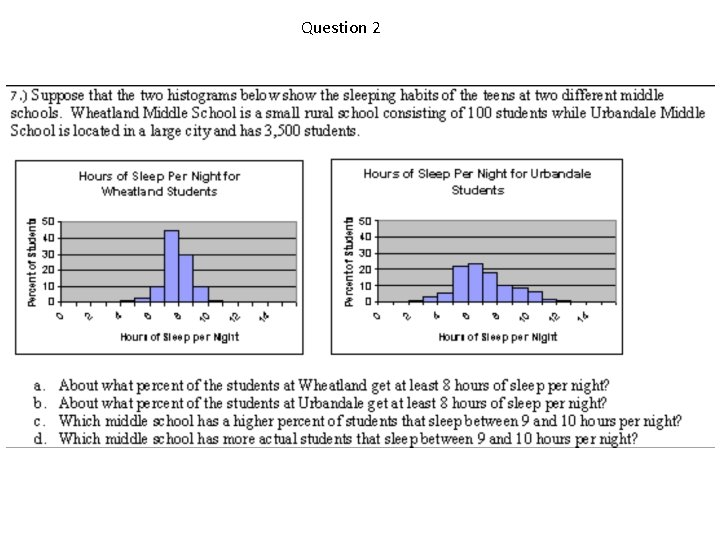Question 2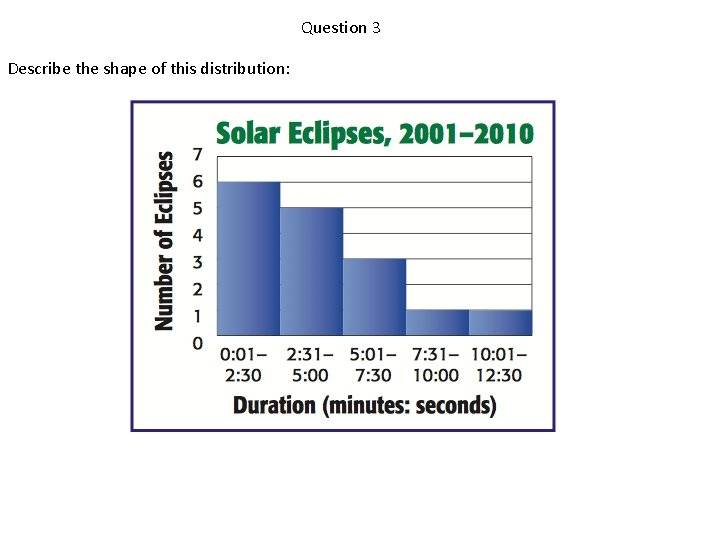Question 3 Describe the shape of this distribution:Question 4Question 5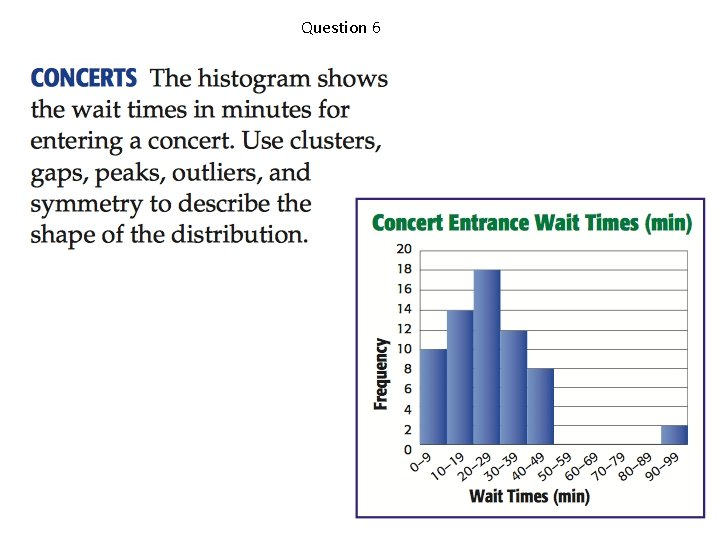Question 6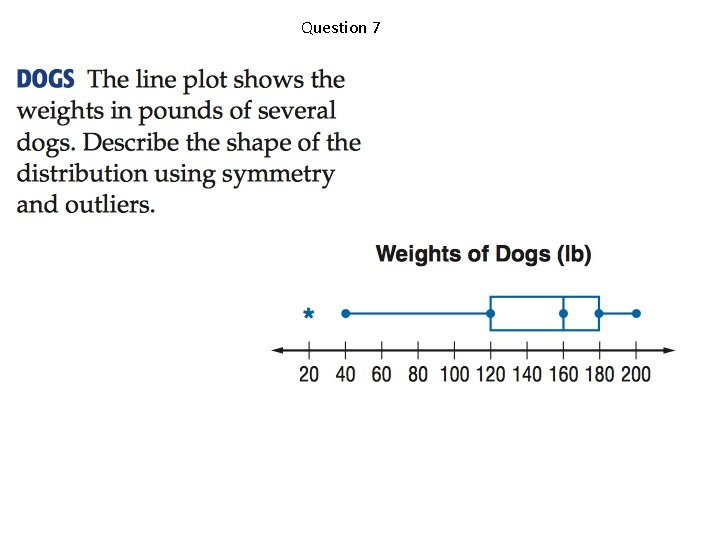Question 7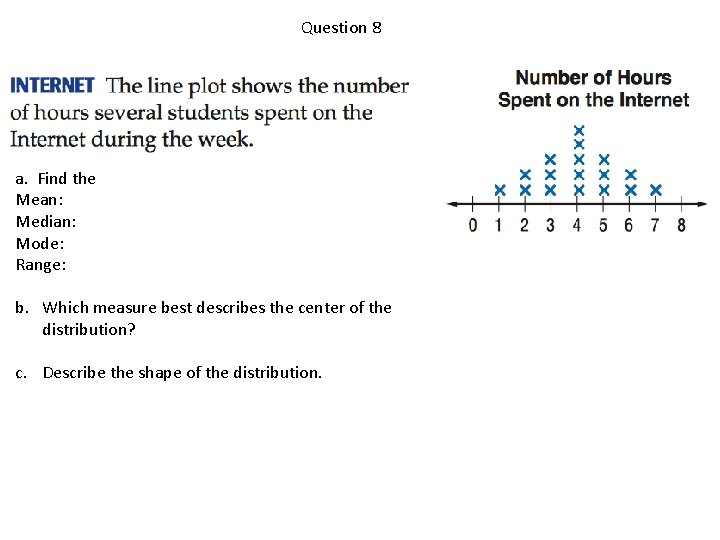Question 8 a. Find the Mean: Median: Mode: Range: b. Which measure best describes the center of the distribution? c. Describe the shape of the distribution.Question 9 Use clusters, gaps, peaks, outliers, and symmetry to describe the shape of the distribution.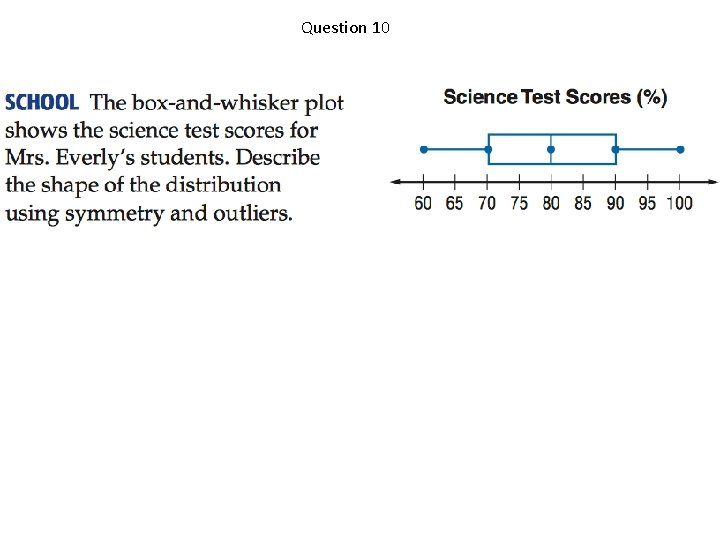Question 10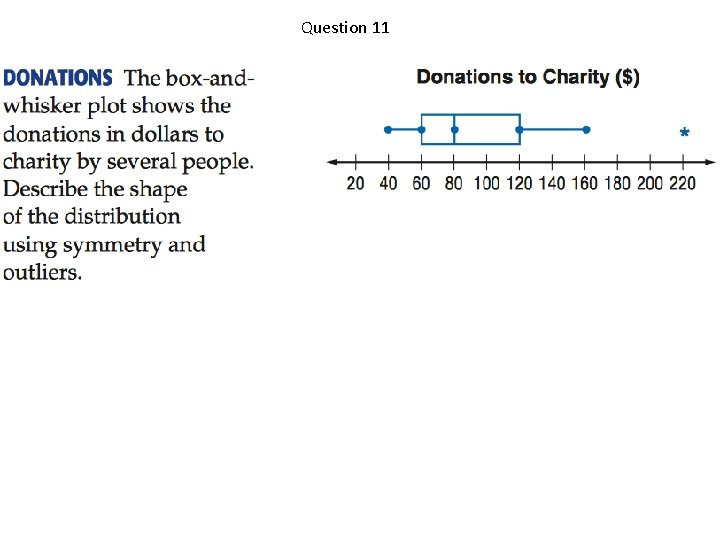Question 11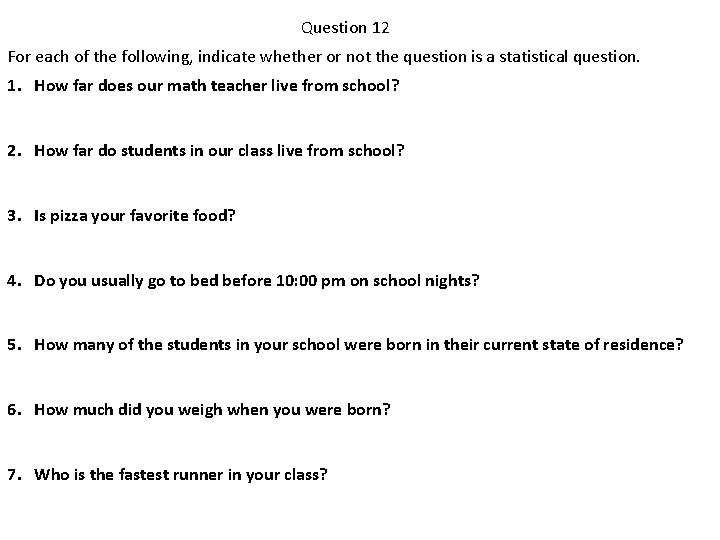Question 12 For each of the following, indicate whether or not the question is a statistical question. 1. How far does our math teacher live from school? 2. How far do students in our class live from school? 3. Is pizza your favorite food? 4. Do you usually go to bed before 10: 00 pm on school nights? 5. How many of the students in your school were born in their current state of residence? 6. How much did you weigh when you were born? 7. Who is the fastest runner in your class?Question 13 Identify the measures of variability. What do they tell you about the data?Question 14 Identify the measures of center and explain how you find each one.Question 15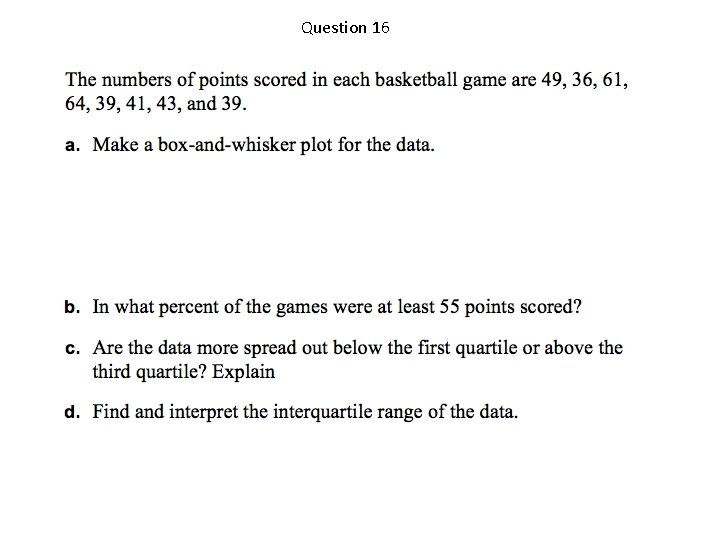Question 16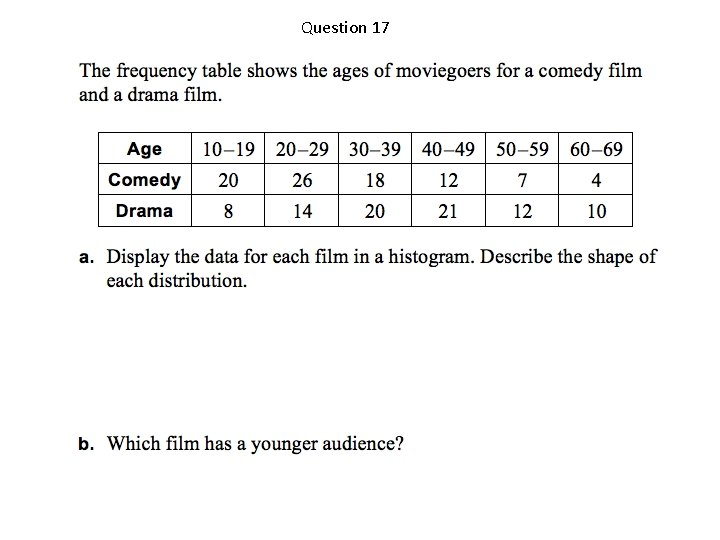Question 17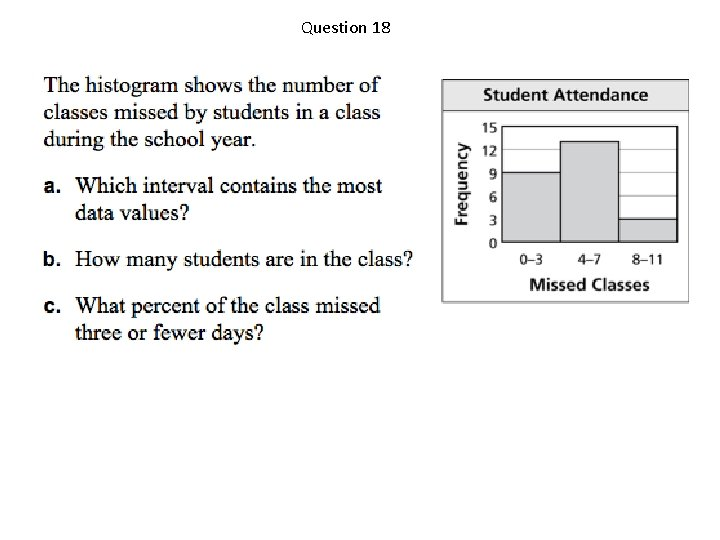Question 18Question 19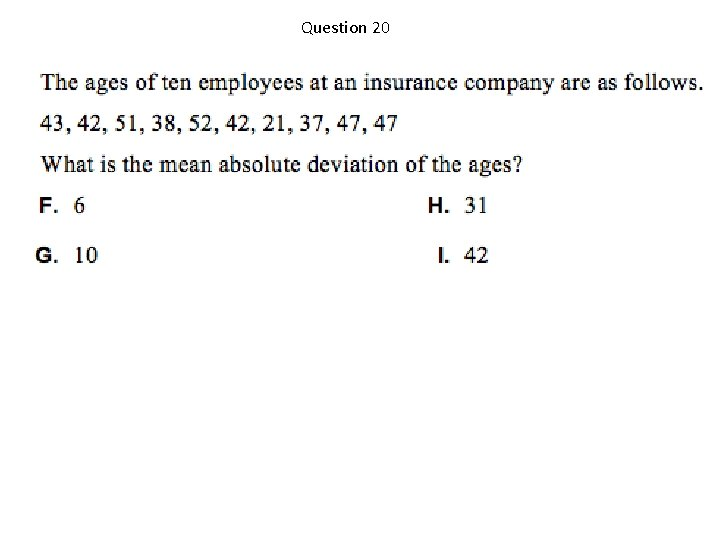Question 20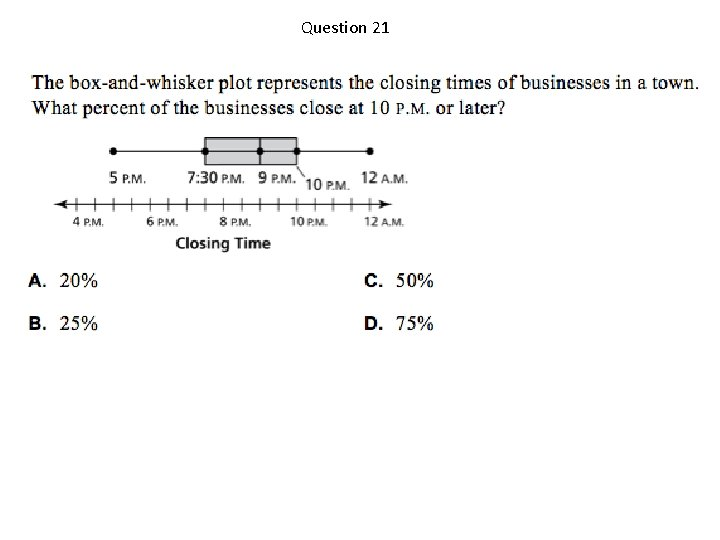Question 21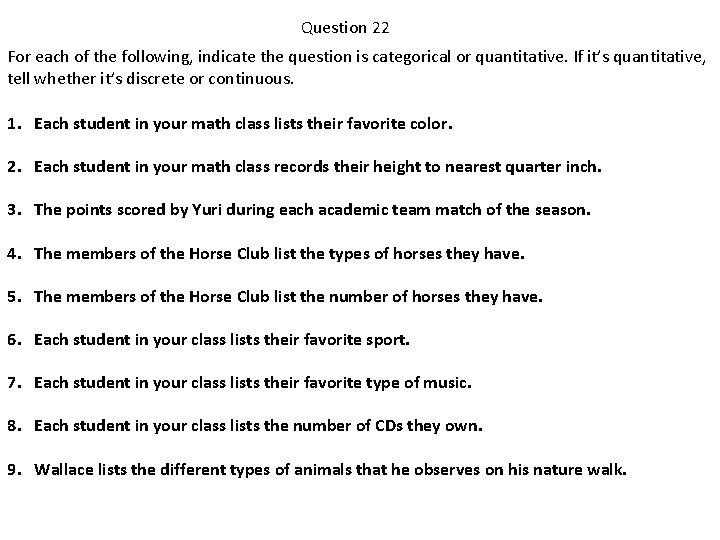Question 22 For each of the following, indicate the question is categorical or quantitative. If it’s quantitative, tell whether it’s discrete or continuous. 1. Each student in your math class lists their favorite color. 2. Each student in your math class records their height to nearest quarter inch. 3. The points scored by Yuri during each academic team match of the season. 4. The members of the Horse Club list the types of horses they have. 5. The members of the Horse Club list the number of horses they have. 6. Each student in your class lists their favorite sport. 7. Each student in your class lists their favorite type of music. 8. Each student in your class lists the number of CDs they own. 9. Wallace lists the different types of animals that he observes on his nature walk.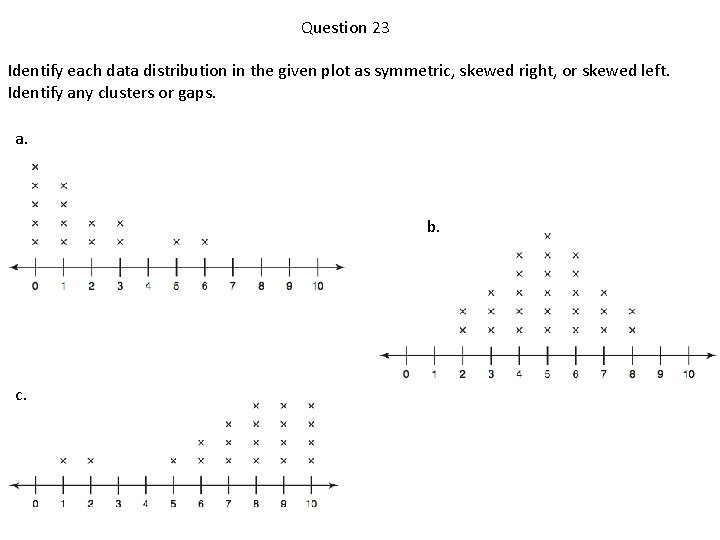Question 23 Identify each data distribution in the given plot as symmetric, skewed right, or skewed left. Identify any clusters or gaps. a. b. c.Question 24 a. During which 3 -month interval did Really Realty sell the most homes, and how many homes did they sell during that interval? b. How many homes did Really Realty sell in 2009?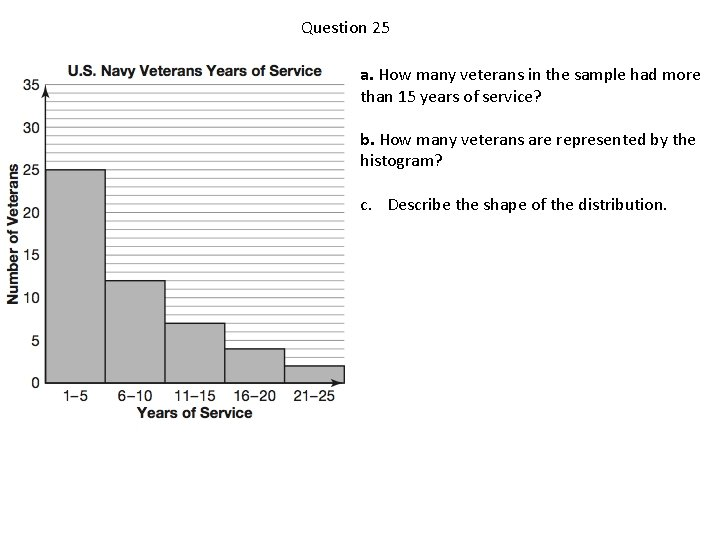Question 25 a. How many veterans in the sample had more than 15 years of service? b. How many veterans are represented by the histogram? c. Describe the shape of the distribution.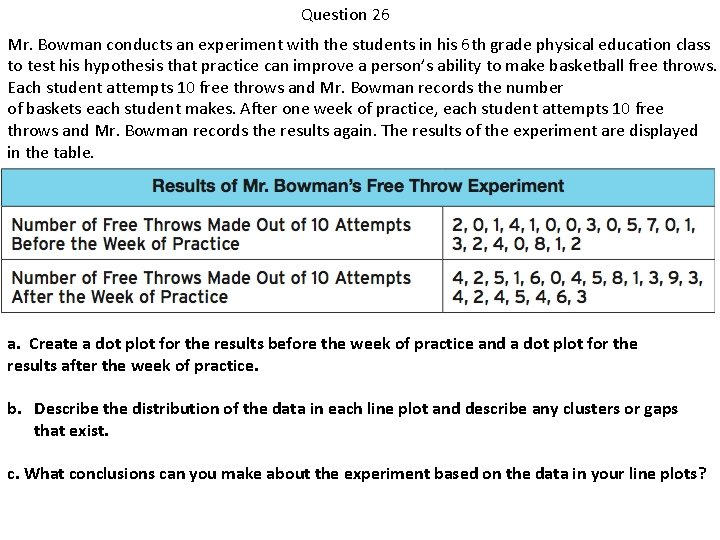Question 26 Mr. Bowman conducts an experiment with the students in his 6 th grade physical education class to test his hypothesis that practice can improve a person’s ability to make basketball free throws. Each student attempts 10 free throws and Mr. Bowman records the number of baskets each student makes. After one week of practice, each student attempts 10 free throws and Mr. Bowman records the results again. The results of the experiment are displayed in the table. a. Create a dot plot for the results before the week of practice and a dot plot for the results after the week of practice. b. Describe the distribution of the data in each line plot and describe any clusters or gaps that exist. c. What conclusions can you make about the experiment based on the data in your line plots?Question 27 Suppose the salaries (in dollars) of six employees are: 8000, 10000, 15000, 16000, 20000 and 39000. a. What are the median and mean salaries? b. Why are they such different numbers? c. Which measure of center is the better pick for this data? Why?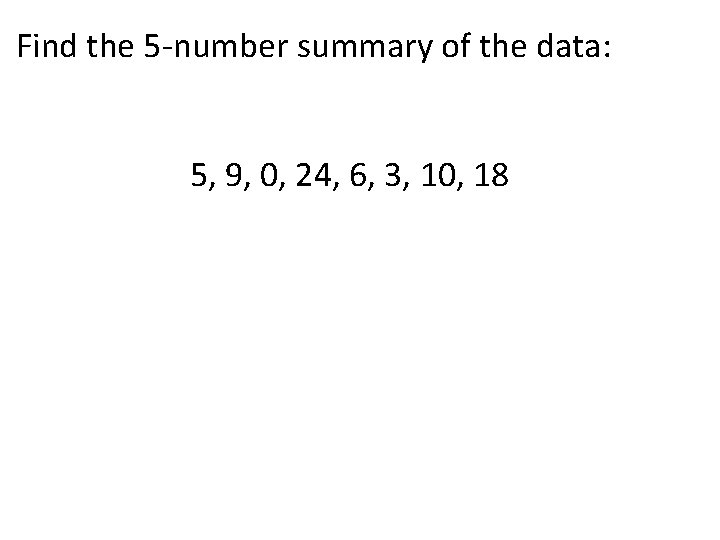Find the 5 -number summary of the data: 5, 9, 0, 24, 6, 3, 10, 18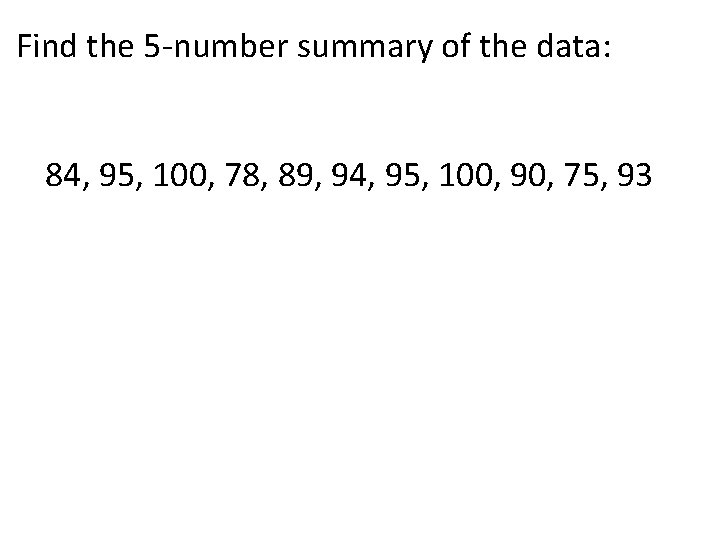Find the 5 -number summary of the data: 84, 95, 100, 78, 89, 94, 95, 100, 90, 75, 93For each set of numbers, determine which measure of center would best describe the data and why: a. 5, 9, 0, 24, 8, 3, 10 b. 88, 92, 100, 81, 79, 95, 85 c. 95, 100, 105, 50, 93, 87, 90, 88,